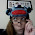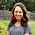## Friday, July 20, 2012

### Factoring cubic binomials

To be finished later, but here is a quick idea that came up:

Factoring binomials of cubes (x^3 + y^3) or (x^3 - y^3)

My mnemonic is "SQuiggy CHases Many Purple SQuirrels." It stands for:

• SQuare (the first term)
• CHange (the sign)
• Multiply (the two terms)
• Plus
• SQuare (the second term)

So, (x^3 + y^3) = (x+y)(x^2 - xy + y^2) and (x^3 - y^3) = (x-y)(x^2 + xy + y^2)

1.SWEET!! In this day and age, where "hey look a squirrel" is a punchline everywhere, this will help so much!

What I've done in the past to help remember the signs:
"what it is...what it isn't...plus" (with gestures like I'm rapping)

So if it's a sum of cubes the first sign is + because that's what it is, the second sign is - because that's what it isn't...plus.

My students didn't have issues remembering the square, multiply, square part...just the signs. So this worked for me.

Thanks for sharing this just in case my future students struggle with all of it.

2.Ooo! Thank you! I usually teach the mnemonic SOAP to remember the signs (Same sign, Opposite sign, Always Positive). But I like this even better!

3.I think if you added REX for Remove EXponents in front of your phrase, then this method would describe the binomial factor that is in front of the trinomial factor. It also would be more memorable since REX is likely the name of a dog and we know most dogs like to chase squirrels. ;-)